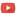KE8QZC | SFW | TSWSyllabus [tex]

Exams
Exam 1: [pdf] [tex]
Exam 2: [pdf] [tex]
Exam 3: [pdf] [tex]

Homework
Homework 1 (due 21 August) (solution): Section 1.1: #11, 12, 17, 18, 31, 32, 33, 34, 38, 39, 40, 41, 43, 44, 45, 46, 47, 48, 52, 53, 54, 55, 57 (a,b)

Homework 2 (due 28 August) (solution): Section 2.1: 1, 4, 5, 6, 11, 12, 27, 28, 29, 30; Section 2.2: 1, 2, 3, 4, 9 (only do part b), 10 (only do part b), 25, 26, 32; Section 2.3: 1, 2, 3, 4, 7, 8, 9, 10, 22, 23, 24, 25, and the following additional problems:
Problem A: Solve the system of equations (field: $\mathbb{C}$) $$\left\{ \begin{array}{llll} (2+i)z_1 & + 3z_2 &= 1 \\ - z_1 &+ iz_2 &= 0 \\ \end{array} \right.$$ note: it is helpful to remember that to "simplify" a complex number of the form $\dfrac{a+bi}{c+di}$ you may multiply by a "convenient form of $1$" defined from the "complex conjugate" of the denominator as follows: $$\dfrac{a+bi}{c+di} = \dfrac{a+bi}{c+di} \dfrac{c-di}{c-di} = \dfrac{(a+bi)(c-di)}{c^2+d^2} = \dfrac{ac + bd}{c^2+d^2} + \dfrac{bc-ad}{c^2+d^2}i.$$ Problem B: Solve the system of equations (field: $\mathbb{Z}_5$) $$\left\{ \begin{array}{lll} 3x_1 &+ 4x_2 &= 1 \\ x_1 &- 2x_2 &= 0 \end{array} \right.$$
Homework 3 (due 6 September) (solution): Section 2.3: 1, 2, 3, 4, 7, 8, 9, 10, 22, 23, 24, 25, 30, 31; Section 3.1: #1, 2, 3, 4 and the following two problems:
Problem A: Determine if the following set of vectors is linearly independent (field: $\mathbb{C}$): $$\left\{ \left[ \begin{array}{ll} 3 \\ -i \\ 2 \end{array} \right], \left[ \begin{array}{ll} 2 \\ i \\ 3 \end{array} \right], \left[ \begin{array}{ll} 1 \\ 1 \\ 1 \end{array} \right] \right\}$$ Problem B: Determine if the following set of vectors is linearly independent (field: $\mathbb{Z}_3$): $$\left\{ \left[ \begin{array}{ll} 1 \\ 2 \\ \end{array} \right], \left[ \begin{array}{ll} 2 \\ 1 \end{array} \right] \right\}$$ Problem C: Determine if the following set of vectors is linearly independent (field: $\mathbb{Z}_5$): $$\left\{ \left[ \begin{array}{ll} 3 \\ 2 \\ 0 \end{array} \right], \left[ \begin{array}{ll} 1 \\ 1 \\ 1 \end{array} \right], \left[ \begin{array}{ll} 1 \\ 2 \\ 1 \end{array} \right] \right\}$$
Homework 4 (due 11 September) (solution): Section 3.1: #5, 6, 7, 8, 9, 10, 17, 22, 36, 40; Section 3.2: #3, 4, 6, 7, 14, 15, 44(b) and the following additional problems:
Problem A: If $A \in \mathbb{Z}_5^{2 \times 2}$ and $B \in \mathbb{Z}_5^{2 \times 2}$ with $$A = \left[ \begin{array}{ll} 1 & 2 \\ 3 & 4 \end{array} \right]$$ and $$B = \left[ \begin{array}{ll} 1 & 3 \\ 0 & 1 \end{array} \right],$$ compute $AB, BA, A^TB^T, B^TA^T,$ and $(BA)^T$. Are any of these matrices related?

Problem B: If $A, B, C \in \mathbb{C}^{2 \times 2}$ with $$A = \left[ \begin{array}{ll} 1 & 1 \\ i & 0 \end{array} \right],$$ $$B = \left[ \begin{array}{ll} 0 & 2+i \\ 1 & 1 \end{array} \right],$$ and $$C = \left[ \begin{array}{ll} -1 & 1+i \\ 1-i & 1 \end{array} \right].$$ Use the definition of linear independence (i.e. the equation) and solve it to determine whether $\{A,B,C\}$ is a linearly independent or linearly dependent set of matrices.
Homework 5 (due 25 September) (solution): see this file

Homework 6 (due 29 September) (solution): Section 3.3: #2, 3, 4, 50, 54 ;Section 6.1 #2, 8, 10, 14, 16, 18, 20, 24, 26, 28, 34, 36

Homework 7 (due 4 October) (solution): Section 6.1: #46,48, 50, 53, 54, 61, 62

Homework 8 (due 11 October) (solution): Section 6.2: #5, 6, 7, 8, 10, 11, 18, 22

Homework 9 (due 18 October) (solution): Section 6.2: #19, 23, 27, 28, 29, 34, 35, 39, 44 (proof by contradiction!)

Homework 10 (due 23 October) (solution): Section 6.3: #1, 2, 6, 7, 9; Section 6.4: #1, 2, 3, 5, 6, 9, 10, 14, 17, 24, 27

Homework 11 (due 30 October) (solution): Section 6.4: #20, 25; Section 6.5: #2, 3, 4, 9, 11, 15, 16, 21, 25, 28, 32

Homework 12 (due 8 November) (solution): Section 6.5: #15, 16, 21, 25, 28, 32; and the following additional problem:

Problem A: ("Laguerre polynomials") Let $T_n \colon \mathscr{P}_2 \rightarrow \mathscr{P}_2$ be defined by $T_n(p)=xp''+(1-x)p'+np$ for $n=0,1,2$. Find $\mathrm{ker}(T_n)$ for $n=0,1,2$.

Homework 13 (due 15 November) (solution): The following homework refers to Examples in the notes on inner products.

Problem A. Let $H=(\mathbb{R}^3,\langle \cdot,\cdot \rangle)$ be the inner product space of Example 1. Let $\vec{x}=\begin{bmatrix} 3 \\ 2\\ -1\end{bmatrix}$ and $\vec{y}=\begin{bmatrix} 2 \\ 1 \\ 17\end{bmatrix}$. Compute $\langle \vec{x},\vec{y} \rangle$.
Problem B. Let $H=(\mathbb{P}, \langle \cdot,\cdot \rangle)$ be the inner product space of Example 2. Let $\vec{p}(x)=x-1$, $\vec{q}(x)=x^2$. Compute both of the inner products $\langle \vec{p},\vec{q} \rangle$ and $\langle \vec{p},\vec{p} \rangle$ using integration by parts.
Problem C. Let $H=(\mathscr{C}[0,1],\langle \cdot,\cdot \rangle)$ be the inner product space of Example 3. Let $f(x)=\log(x+1)$ and $g(x)=1$. Calculate $\langle f,g \rangle$. Let $h_1(x)=x^2$ and $h_2(x)=\sin(x)$. Calculate $\langle h_1,h_2 \rangle$ (hint: use integration by parts or Wolframalpha).
Problem D. Let $H=(\ell^1(\mathbb{R}),\langle\cdot,\cdot\rangle)$ be the inner product space of Example 4. Let $\{a_k\}_{k=0}^{\infty} = \left\{ \dfrac{1}{3^k} \right\}_{k=0}^{\infty}$ and $\{b_k\}_{k=0}^{\infty} = \left\{ \dfrac{1}{7^k} \right\}_{k=0}^{\infty}$. Calculate $\left\langle \left\{ a_k \right\}, \left\{b_k\right\} \right\rangle$ (hint: this is a geometric series). Let $\left\{c_k\right\}=\left\{d_k\right\}=\sqrt{\dfrac{1}{k!}}$. Calculate $\langle c_k,d_k \rangle$ (hint:recall the power series $e^x = \displaystyle\sum_{k=0}^{\infty} \dfrac{x^k}{k!}$).
Problem E. Let $H=(\mathbb{C},\langle\cdot,\cdot\rangle)$ be the inner product space of Example 5. Let $\vec{x}=5+4i$ and $\vec{y}=9-11i$. Compute $\langle \vec{x},\vec{y}\rangle$. Let $z_1=21+16i$ and $z_2=\dfrac{11-5i}{2+i}$. Calculate $\langle z_1,z_2\rangle$ (hint: mutltiply $z_2$ by $1=\dfrac{2-i}{2-i}$ to put $z_2$ into the form $z_2=a+bi$; this is similar to "rationalizing denominators").
Problem F.: Let $(\mathbb{R}^{4 \times 1},\langle \vec{x},\vec{y} \rangle)$ be an inner product space where $\langle \vec{x},\vec{y} \rangle$ denotes dot product.
Show that the vectors $\vec{a}=\begin{bmatrix} 1 \\ 2 \\ 3 \\ 4 \end{bmatrix}$ and $\vec{b}=\begin{bmatrix} -4 \\ -3 \\ 2 \\ 1 \end{bmatrix}$ are orthogonal vectors.
Problem G.: Show that set $\left\{ \begin{bmatrix} 1 \\ 0 \\ 0 \\ 0 \end{bmatrix}, \begin{bmatrix} 0 \\ 1 \\ 0 \\ 0 \end{bmatrix}, \begin{bmatrix} 0 \\ 0\\ 1 \\ 0 \end{bmatrix}, \begin{bmatrix} 0 \\ 0 \\ 0 \\ 1 \end{bmatrix} \right\}$ is a mutually orthogonal set of vectors. Also show that the set $\left\{ \begin{bmatrix} 1 \\ 1 \\ 0 \\ 0 \end{bmatrix}, \begin{bmatrix} 0 \\ 1 \\ 1 \\ 0 \end{bmatrix}, \begin{bmatrix} 0 \\ 0 \\ 1 \\ 0 \end{bmatrix}, \begin{bmatrix} 0 \\ 0 \\ 0 \\ 1 \end{bmatrix} \right\}$ is not a mutually orthogonal set of vectors.
Problem H.: Consider the vector space $(\mathbb{P},\langle \cdot,\cdot \rangle)$ where the inner product is given by $$\langle p(x),q(x) \rangle = \displaystyle\int_{-\infty}^{\infty} p(x)q(x)e^{-x^2} dx.$$ It can be shown (via methods of calculus 2) that the moments in this inner product space are $$\langle 1,1 \rangle=\sqrt{\pi},$$ $$\langle x,1 \rangle=0,$$ $$\langle x^2,1 \rangle=\dfrac{\sqrt{\pi}}{2},$$ $$\langle x^3,1 \rangle = 0,$$ $$\langle x^4,1 \rangle = \dfrac{3\sqrt{\pi}}{4},$$ $$\langle x^5,1 \rangle = 0,$$ $$\langle x^6,1 \rangle = \dfrac{15\sqrt{\pi}}{8}.$$ Use these moments and the "linear in the first argument" property of inner products to compute $\langle 4x^2+3x+9,1\rangle$ and $\langle 32x^5-64x^3+24x,1\rangle$.
Problem I.: Consider the inner product space $(C[0,1],\langle \cdot,\cdot \rangle)$ where $$\langle f,g \rangle = \displaystyle\int_0^1 f(x)g(x)x^2 dx.$$ Compute $\mathrm{proj}_{x^2-3x} (5x+2)$ and $\mathrm{proj}_{5x+2}(x^2-3x)$.
Problem J.: Consider the inner product space $(\mathbb{R}^{3\times 1},\langle \cdot,\cdot \rangle)$, where $\langle \cdot,\cdot \rangle$ denotes the dot-product. Consider the set $\{v_1,v_2,v_3\}$ where $v_1=\begin{bmatrix} 1 \\ 1 \\ 1 \end{bmatrix}, \vec{v}_2 = \begin{bmatrix} 2 \\ 4 \\ 8 \end{bmatrix}, \vec{v}_3 = \begin{bmatrix} 3 \\ 9 \\ 27 \end{bmatrix}$. It is clear that this set is not an orthogonal set of vectors. Apply the Gram-Schmidt process to orthogonalize this set.
Problem K.: Consider the inner product space $(\mathbb{P},\langle \cdot,\cdot \rangle)$ where $\langle \vec{p},\vec{q} \rangle = \displaystyle\int_{-1}^1 \vec{p}(x)\vec{q}(x) dx$. Apply the Gram-Schmidt process to the sequence $(x^n)_{n=0}^{\infty}$ to find the first four polynomials orthogonal with respect to $\langle \cdot,\cdot \rangle$. (note: these polynomials are called Legendre polynomials)